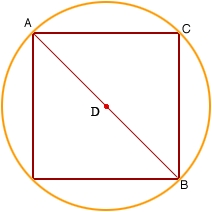# Calculate 8252

Calculate in cm2 the area of a circle whose diameter is equal to the length of the diagonal of a square ABCD with a side of 4cm.

S =  25.1327 cm2

### Step-by-step explanation:Did you find an error or inaccuracy? Feel free to write us. Thank you!

Tips for related online calculators
The Pythagorean theorem is the base for the right triangle calculator.